# Introduction to Python – Data Analysis

Weather is something we all experience. Which is why you’ll often find weather-related data used in data analysis courses. Of course, as oceanographers, weather data is far more relevant to our research goals, but it’s also useful to start with more accessible weather or “ocean weather” related examples, as those will be more familiar with students, before diving into more niche oceanographic datasets.

That’s why I love the NDBC dataset, because it makes weather data easily accessible. This allows students to visualize data and look for patterns they are hopefully familiar with (i.e. the weather near them), while they are also learning new data analysis techniques and developing their programming skills.

Here is just one example of showing the annual cycle of Sea Surface Temperature in the Mid Atlantic at NDBC Station 44025 (my favorite station – everyone should have one ;).With 10-years of data plotted at once, you can quickly see what the mean and variability look like over the course of the year, as well as the impact from the occasional extreme event (read: storm).

With larger datasets like NDBC, which has stations all over the world, students can compare the patterns they’ve identified and are familiar with, with patterns they may not be as familiar with. This could include weather in other areas of the world, or new processes that they are learning about, such as wind/wave correlations, sea breezes (land/air interactions), or heat capacity (air/sea temp relationships).

As part of our 2020 Virtual REU, I created the following notebook to demonstrate some basic data analysis techniques using a few years of data from NDBC Station 44025. This notebook is not comprehensive (that would require a longer course or a textbook to cover), but you can consider it a hodgepodge sampling of some of the most common techniques one might see for this kind of timeseries dataset.

Plus, what I think makes this notebook so cool, is that it demonstrates that it doesn’t take much code to make these sorts of graphs, thanks to the awesome pandas library in python.

Here are a few of the data analysis techniques I highlight in the notebook:

• Basic Statistics – Including count, mean, std, min, max and percentile calculations, as well as identifying extreme values.
• Histograms – An important first step in understanding the shape of a dataset. Along with the basic statistics, it provides a pictorial representation that is quick to interpret.
• Running Averages – Often “raw” data is too “noisy” for how you want to use it. Thankfully, you can easily use .resample() in pandas to calculate hourly, daily or monthly averages (or indeed, any interval you like) to smooth things out.
• Daily Cycle – Many processes repeat regularly over the course of a day. (Hello sunlight!) You can use .groupby() in pandas to average data by the hour of the day to see if there is a diurnal cycle. However, you need to be careful to look at both the mean and the variability about that mean to see if it’s meaningful. In addition, this cycle may differ over the course of a year, so you may also want to look at these averages by season or month or year as well.
• Annual Cycle by Month or Day – Is there an annual pattern? You can also use .groupby() to average your dataset by month or yearday. This will calculate an annual cycle for one year or many years.
• Inter-annual Variation and Variability – Understanding the daily or annual pattern is a great first step, but if you have a longer dataset, you will probably want to investigate how this pattern changes from year to year. Using more complex .groupby() commands or box plots you can analyze: How much variability is there? Is there a seasonal trend that is increasing or decreasing? Is the mean or variability dependent on the year, month, or season? This is when things start to get fun.
• Plot the Raw Data! – When calculating averages, it’s always important to plot the raw data as well as a check, so you can see the averages (and standard deviations or other percentiles about them) in context. It’s important to make sure your data makes sense, without any outliers biasing the averages.
• Wind Roses – If your data is 2-dimenstional, as wind data is, wind roses are a great way to show the directional relationship in your data. Basically they’re a 2-dimensional histogram, common in meteorology, but a bit tricky to create. Thankfully, there’s a library for that too!
• Anomaly Plots – Once you have a long-term timeseries dataset (which NDBC has at many locations) you can calculate a climatology, which is long-term average. You can then use the climatology to calculate anomalies, which are differences from the long-term mean. This is a concept that is often not as familiar with students, and so going through the calculation is a great way to develop their comfort in interpreting anomaly datasets.

This summer, we didn’t spend too much time on this notebook in our mini-research workshop. But having this set of examples handy, made it easy for students to refer to when developing their own data analysis projects.  Most students incorporated one or two of the examples, depending on their goals.

I’m also happy I had a chance (excuse) to put these examples together, because as you can see, there is so much you can do with just a few lines of code. And there is so much more you can do in the wide-ranging field of timeseries data analysis, that we’ve barely scratched the surface, even after 3 notebooks.

I hope you find this notebook helpful as you develop your own activities.

This post is part of our 2020 Summer REU Intro to Python series. See also Part 1, Part 2, and Part 4.

# Activity 3 - Data Analysis¶

2020 Data Labs REU

Written by Sage Lichtenwalner, Rutgers University, June 12, 2020

In this notebook we will demonstrate a few data analysis strategies you can use to analyze a timeseries dataset. This is by no means an exhaustive list, but hopefully enough to get you started with some common strategies.

The examples today will continue to use the mooring and shore station timeseries data available from NDBC.

In :
```# Notebook setup
import xarray as xr
!pip install netcdf4

import matplotlib.pyplot as plt
import numpy as np

# Let's make our plots pretty
import seaborn as sns
sns.set()
```
```Requirement already satisfied: netcdf4 in /usr/local/lib/python3.6/dist-packages (1.5.3)
Requirement already satisfied: numpy>=1.7 in /usr/local/lib/python3.6/dist-packages (from netcdf4) (1.18.5)
Requirement already satisfied: cftime in /usr/local/lib/python3.6/dist-packages (from netcdf4) (1.1.3)
```
```/usr/local/lib/python3.6/dist-packages/statsmodels/tools/_testing.py:19: FutureWarning: pandas.util.testing is deprecated. Use the functions in the public API at pandas.testing instead.
import pandas.util.testing as tm
```

## Load a NDBC Dataset using Pandas¶

Following our previous examples, let's load some data from NDBC and convert it to a Pandas Dataframe.

In :
```# Open dataset
ds = xr.open_dataset('https://dods.ndbc.noaa.gov/thredds/dodsC/data/stdmet/44025/44025.ncml')

# Subset the dataset to 1 year
ds = ds.sel(time=slice('2019-01-01','2020-01-01'))

# Convert to Pandas Dataframe
df = ds.to_dataframe().reset_index().set_index('time')
```

How big is our dataset?

In :
```df.shape
```
Out:
`(8669, 15)`

Once we have data loaded in Pandas, we can use `.head()` `.tail()` and `.sample()` to see what that dataset looks like. You can pass a number to any of these functions to print out a specific number of rows.

In :
```df.head(3)
```
Out:
latitude longitude wind_dir wind_spd gust wave_height dominant_wpd average_wpd mean_wave_dir air_pressure air_temperature sea_surface_temperature dewpt_temperature visibility water_level
time
2019-01-01 00:50:00 40.250999 -73.164001 135.0 11.3 14.1 1.56 00:00:05.559999 00:00:04.710000 153.0 1018.400024 8.1 7.1 NaN NaN NaN
2019-01-01 01:50:00 40.250999 -73.164001 127.0 11.2 14.0 1.92 00:00:05.880000 00:00:05.090000 135.0 1016.599976 8.3 7.1 NaN NaN NaN
2019-01-01 02:50:00 40.250999 -73.164001 137.0 12.7 15.3 2.23 00:00:06.250000 00:00:05.219999 143.0 1013.599976 8.7 7.2 NaN NaN NaN

This also works on specific columns.

In :
```# One column
```
Out:
```time
2019-01-01 00:50:00    8.1
2019-01-01 01:50:00    8.3
2019-01-01 02:50:00    8.7
Name: air_temperature, dtype: float32```
In :
```# Multiple columns
```
Out:
longitude latitude
time
2019-01-01 00:50:00 -73.164001 40.250999
2019-01-01 01:50:00 -73.164001 40.250999
2019-01-01 02:50:00 -73.164001 40.250999

## Basic Bulk Statistics¶

Thanks to the magic of pandas, we can quickly calculate a bunch of statistics on our dataset using the `.describe()` function.

In :
```df.describe()
```
Out:
latitude longitude wind_dir wind_spd gust wave_height dominant_wpd average_wpd mean_wave_dir air_pressure air_temperature sea_surface_temperature dewpt_temperature visibility water_level
count 8669.000000 8669.000000 8642.000000 8669.000000 8669.000000 8660.000000 8641 8660 8641.000000 8669.000000 8669.000000 8669.000000 0.0 0.0 0.0
mean 40.250999 -73.164001 195.684679 6.693528 8.168374 1.274927 0 days 00:00:07.330060 0 days 00:00:04.976726 162.821548 1016.819458 12.502096 13.550716 NaN NaN NaN
std 0.000000 0.000000 100.992567 3.437403 4.219831 0.736393 0 days 00:00:02.633673 0 days 00:00:00.979788 70.678750 8.206482 8.092100 6.862940 NaN NaN NaN
min 40.250999 -73.164001 1.000000 0.000000 0.000000 0.220000 0 days 00:00:02.470000 0 days 00:00:02.950000 1.000000 985.599976 -11.700000 3.100000 NaN NaN NaN
25% 40.250999 -73.164001 106.250000 4.200000 5.100000 0.760000 0 days 00:00:05.260000 0 days 00:00:04.260000 110.000000 1011.500000 6.100000 6.900000 NaN NaN NaN
50% 40.250999 -73.164001 213.000000 6.200000 7.400000 1.080000 0 days 00:00:07.139999 0 days 00:00:04.829999 152.000000 1016.900024 12.000000 13.100000 NaN NaN NaN
75% 40.250999 -73.164001 282.000000 8.700000 10.600000 1.570000 0 days 00:00:09.090000 0 days 00:00:05.559999 194.000000 1022.299988 20.100000 20.299999 NaN NaN NaN
max 40.250999 -73.164001 360.000000 20.299999 26.900000 4.600000 0 days 00:00:17.389999 0 days 00:00:09.739999 360.000000 1040.599976 27.299999 26.600000 NaN NaN NaN
In :
```# For specific columns
df[['air_temperature','sea_surface_temperature','wind_spd']].describe()
```
Out:
air_temperature sea_surface_temperature wind_spd
count 8669.000000 8669.000000 8669.000000
mean 12.502096 13.550716 6.693528
std 8.092100 6.862940 3.437403
min -11.700000 3.100000 0.000000
25% 6.100000 6.900000 4.200000
50% 12.000000 13.100000 6.200000
75% 20.100000 20.299999 8.700000
max 27.299999 26.600000 20.299999

As with a regular Pandas dataframe, these statistics are also a dataframe that can be exported to CSV, in case we want to use it elsewhere.

Note, just because it saves a lot of digits, that doesn't mean they're significant!

In :
```# Saving statistics
df[['air_temperature','sea_surface_temperature','wind_spd']].describe().to_csv()
```
Out:
`',air_temperature,sea_surface_temperature,wind_spd\ncount,8669.0,8669.0,8669.0\nmean,12.502096176147461,13.550716400146484,6.693528175354004\nstd,8.092100143432617,6.862939834594727,3.4374029636383057\nmin,-11.699999809265137,3.0999999046325684,0.0\n25%,6.099999904632568,6.900000095367432,4.199999809265137\n50%,12.0,13.100000381469727,6.199999809265137\n75%,20.100000381469727,20.299999237060547,8.699999809265137\nmax,27.299999237060547,26.600000381469727,20.299999237060547\n'`

### Individual statistics¶

We can also request these calculations separately.

• count() - Number of values
• mean()
• std() - Standard Deviation
• min()
• max()
• quantile() - Percentile, defaults to 0.5 = 50%
In :
```# Example statistical calc
df.mean()
```
Out:
```latitude                                   40.251
longitude                                 -73.164
wind_dir                                  195.685
wind_spd                                  6.69353
gust                                      8.16837
wave_height                               1.27493
dominant_wpd               0 days 00:00:07.330060
average_wpd                0 days 00:00:04.976726
mean_wave_dir                             162.822
air_pressure                              1016.82
air_temperature                           12.5021
sea_surface_temperature                   13.5507
dewpt_temperature                             NaN
visibility                                    NaN
water_level                                   NaN
dtype: object```
In :
```# Or we could output these individually
print(round(df['wind_spd'].mean(),2))
print(round(df['wind_spd'].std(),2))
```
```6.69
3.44
```
In :
```# Quickplot to compare
df.wind_spd.plot();
```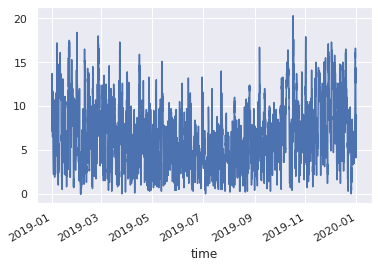In :
```# Quickplot to compare
df.wind_spd.hist(bins=25);
```In :
```# Your turn - Calculate and compare the mean and std for air & water temps
```

### Finding Extremes¶

We can also grab the largest or smallest values in at dataset using `nlargest()` or `nsmallest()`.

In :
```df.wind_spd.nlargest()
```
Out:
```time
2019-10-17 03:50:00    20.299999
2019-10-17 00:50:00    19.799999
2019-10-17 04:50:00    18.900000
2019-10-17 05:50:00    18.900000
2019-01-31 00:50:00    18.400000
Name: wind_spd, dtype: float32```

## Running Averages (resample)¶

We can also easily calculate hourly, daily and monthly averages using pandas.resample. A number of offset options are available for specifying the interval.

Here's a quick example that shows a few different intervals.

In :
```fig, ax = plt.subplots()
fig.set_size_inches(12, 6)
df['sea_surface_temperature'].plot(ax=ax,label='Raw', linestyle='None', marker='.', markersize=1)
df['sea_surface_temperature'].resample('D').mean().plot(ax=ax,label='Daily')
df['sea_surface_temperature'].resample('5D').mean().plot(ax=ax,label='5 Day')
df['sea_surface_temperature'].resample('MS').mean().plot(ax=ax,label='Monthly',marker='d') #MS=Month Start
plt.legend();
```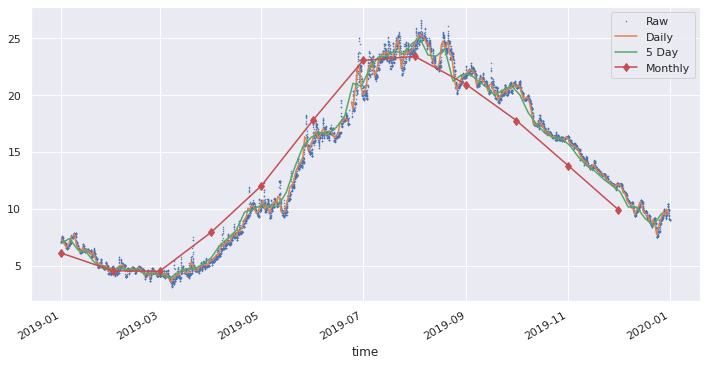Note that by default, pandas only provides Month Start (MS) or Month End (M) periods. That might be find for business, but in oceanography we want our graphs to have physical meaning. One option, that gets us a little closer to a centered average is to use the loffset parameter.

In :
```# Let's try this again, but add a 15-day offset to the monthly average

from datetime import timedelta

fig, ax = plt.subplots()
fig.set_size_inches(12, 6)
df['sea_surface_temperature'].plot(ax=ax,label='Raw', linestyle='None', marker='.', markersize=1)
df['sea_surface_temperature'].resample('MS').mean().plot(ax=ax,label='Month Start',marker='d') #MS=Month Start
df['sea_surface_temperature'].resample('MS',loffset=timedelta(days=15)).mean().plot(ax=ax,label='15th of the Month',marker='d')
plt.legend();
```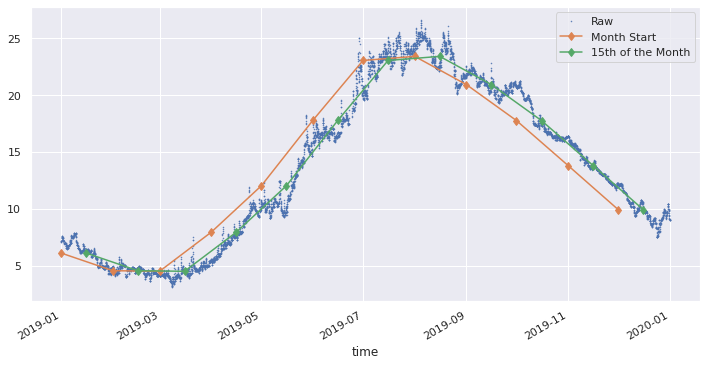In :
```# Your Turn - Plot daily or monthly averages (mean) of Air Temp or Wind Speed
```
In :
```# Your Turn - Plot daily or monthly standard deviation (std) for Air Temp or Wind Speed
```

## Daily & Annual Variation (groupby)¶

Similarly, we can look at process that vary of the course of a day or a year by averaging all the hours of a day or days of a year together. (Days of a month probably wouldn't make much sense in oceanography.) To do this, we can use pandas.groupby.

The following example was inspired by this Seasonal Cycle and Anomaly at NDBC 44025 post.

To see some other cool examples on how groupby() can be used, check out this lesson from Ryan Abernathey.

In :
```# Load 10 years of data
# Open dataset
ds = xr.open_dataset('https://dods.ndbc.noaa.gov/thredds/dodsC/data/stdmet/44025/44025.ncml')

# Subset the dataset to 10 years
ds = ds.sel(time=slice('2010-01-01','2020-01-01'))

# Convert to Pandas Dataframe
df = ds.to_dataframe().reset_index().set_index('time')
```
In :
```# Quickplot of temperature
df['sea_surface_temperature'].plot();
```We can look at this dataset a number of different ways:

• Hour of the day - Is there a diurnal cycle?
• We could also investigate how this changes seasonally, but we won't get into that here.
• Day of the week - Not really useful here
• Day of the month - Also not useful
• Month of the year - Is there an annual pattern?
• Day of the year - This is basically a higher resolution view of the monthly averages.

For ease of use, let's add some additional columns to our dataset with the hour, month and yearday. This isn't strictly needed, we could just use the references directly, e.g. df.index.hour, instead of the simpler df.hour once it's defined. But sometimes it can come in handy.

In :
```# Add column for hour of day
df['hour'] = df.index.hour

# And month
df['month'] = df.index.month

# And day of year
df['yearday'] = df.index.dayofyear
```

### Daily Cycle¶

In :
```# Calculate the hourly mean
hourly_sst = df.sea_surface_temperature.groupby(df.hour).mean()
```
In :
```# Quick plot
hourly_sst.plot();
```That's a nice looking plot with a noticeable diurnal signal, but it's important to notice how small it is (about a half a degree). Remember, we're averaging 10-years of data here. So on average, the hour-by-hour mean is not that far off from the total dataset mean.

We can get a clearer look at this by visualizing the range of the dataset. Let's calculate the quartiles (25, 50, 75%) to see what they look like.

In :
```# Quantile Function Definitions
def q1(x):
return x.quantile(0.25)

def q2(x):
return x.median()

def q3(x):
return x.quantile(0.75)
```
In :
```# Calculate the hourly quartiles and mean
hourly_sst = df.sea_surface_temperature.groupby(df.hour).agg([q1, q2, q3, np.mean])
```
In :
```# Quick plot
hourly_sst.plot();
```So, yeah, this doesn't tell us much, other than the fact there is a wide spread of the data.

This might be a useful analysis if we were looking at a shorter time period (perhaps a week or a month of data), or if we were looking at this question seasonally (say by looking at just Januaries).

### Annual Cycle by Month¶

In :
```# Calculate the average
monthly_sst = df.sea_surface_temperature.groupby(df.month).agg([q1, q2, q3, np.mean])

# Quick Plot
monthly_sst.plot();
```With a long enough dataset, this approach can give us a Monthly Climatology. Here's a quick example which we could also export and save to use elsewhere.

In :
```# Monthly climatology
monthly_climatology = df.groupby(df.index.month).mean()
monthly_climatology
```
Out:
latitude longitude wind_dir wind_spd gust wave_height mean_wave_dir air_pressure air_temperature sea_surface_temperature dewpt_temperature visibility water_level hour month yearday
time
1 40.250999 -73.164001 227.675220 8.371599 10.385938 1.499277 195.126355 1017.010071 2.899611 6.982348 -2.487602 NaN NaN 11.490255 1.0 16.035532
2 40.250999 -73.164001 222.490004 8.024861 9.798648 1.473149 183.770847 1016.210510 3.516074 5.211267 -1.869530 NaN NaN 11.512842 2.0 45.627593
3 40.250999 -73.164001 201.836573 7.574954 9.183776 1.457474 164.837581 1016.639893 4.993104 5.187761 1.684024 NaN NaN 11.507434 3.0 75.218952
4 40.250999 -73.164001 192.415190 6.253114 7.505553 1.341027 156.084502 1015.159424 8.593872 7.763681 5.472376 NaN NaN 11.506242 4.0 106.077598
5 40.250999 -73.164001 173.683474 5.379606 6.379094 1.138626 149.810407 1016.031677 13.113023 12.178101 11.714257 NaN NaN 11.492474 5.0 136.166616
6 40.250999 -73.164001 188.223616 5.222440 6.203795 0.990192 154.321872 1013.318787 18.731003 18.034389 16.534309 NaN NaN 11.488631 6.0 166.646542
7 40.250999 -73.164001 192.705622 4.964872 5.911243 0.937967 157.869180 1014.778870 23.350443 23.085400 20.713778 NaN NaN 11.473254 7.0 197.122998
8 40.250999 -73.164001 187.551736 5.224868 6.250377 0.953593 149.328885 1015.773560 23.589617 23.723413 19.803507 NaN NaN 11.513504 8.0 228.691512
9 40.250999 -73.164001 167.166559 6.106710 7.435549 1.274251 138.031492 1017.589844 20.553637 21.334000 15.588165 NaN NaN 11.522691 9.0 259.099292
10 40.250999 -73.164001 188.024765 7.641332 9.342347 1.477951 160.152623 1016.176208 16.063944 17.821596 11.289342 NaN NaN 11.479465 10.0 288.908168
11 40.250999 -73.164001 214.890794 8.026984 9.975965 1.440345 180.407674 1018.270813 10.397175 13.851008 4.682261 NaN NaN 11.502698 11.0 320.037778
12 40.250999 -73.164001 225.115094 8.126250 10.132651 1.468057 183.294153 1017.480408 6.683538 10.503538 1.401030 NaN NaN 11.504798 12.0 350.223238

### Annual Cycle by Day¶

Finally, let's repeat what we did above, but rather than taking the average for each month, we will take the average for each day, which should result in a "higher resolution" visualization.

In :
```# Calculate the average
daily_sst = df.sea_surface_temperature.groupby(df.yearday).agg([q1, q2, q3, np.mean])

# Quick Plot
daily_sst.plot();
```In :
```# Pretty plot of data by Yearday
plt.figure(figsize=(10,8))
plt.plot(df.yearday,df.sea_surface_temperature,'.',markersize=1,label='Raw Hourly Data');
plt.plot(daily_sst.index,daily_sst['mean'], linewidth=3,label='10 Year Average')

plt.legend()
plt.xlabel('Day of Year')
plt.ylabel('Sea Surface Temperature (C)')
plt.title('Seasonal Cycle of Sea Surface Temperature at NDBC 44025 from 2010-2019');
plt.savefig('NDBC_44025_Seasonal_SST.png');
```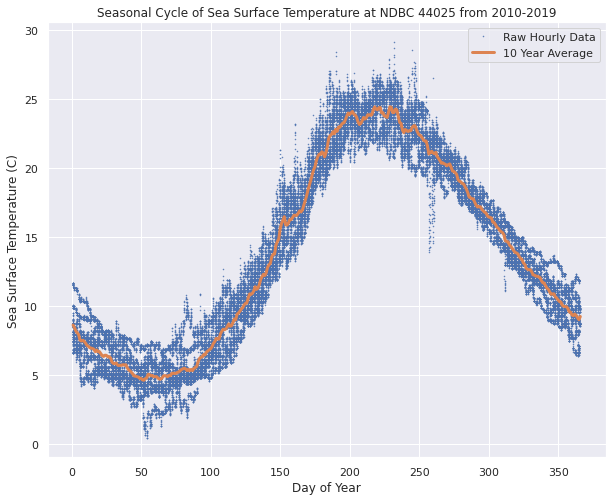## Monthly Averages Plotted by Year¶

Before we use the resample function to calculate a monthly average.

In :
```df['sea_surface_temperature'].resample('MS').mean().plot();
```But what if we want to plot all the years on top of each other to compare the annual cycles? For this, we can use a 2-level groupby.

In :
```a = df.groupby([df.index.year,df.index.month]).mean()
a
```
Out:
latitude longitude wind_dir wind_spd gust wave_height mean_wave_dir air_pressure air_temperature sea_surface_temperature dewpt_temperature visibility water_level hour month yearday
time time
2010 1 40.250999 -73.164001 238.379217 8.622267 10.640351 1.513112 203.136671 1013.670715 2.059379 6.923212 -3.399460 NaN NaN 11.461538 1.0 16.048583
2 40.250999 -73.164001 242.919643 9.318899 11.353422 1.712177 205.031627 1007.152527 1.942559 4.642411 -3.105803 NaN NaN 11.500000 2.0 45.500000
3 40.250999 -73.164001 160.803499 6.812517 8.203095 1.731146 127.974394 1010.653687 5.874697 4.811036 2.790982 NaN NaN 11.512786 3.0 75.002692
4 40.250999 -73.164001 212.004167 5.466528 6.477639 1.042458 156.680168 1013.198608 9.747361 8.782500 6.580000 NaN NaN 11.500000 4.0 105.500000
5 40.250999 -73.164001 194.586022 5.487231 6.500134 1.149220 169.452092 1015.599854 13.488709 12.300673 10.946506 NaN NaN 11.500000 5.0 136.000000
... ... ... ... ... ... ... ... ... ... ... ... ... ... ... ... ... ...
2019 8 40.250999 -73.164001 177.771930 5.117949 6.120783 0.964777 141.256410 1014.819275 23.287720 23.425100 NaN NaN NaN 11.507422 8.0 228.026991
9 40.250999 -73.164001 166.248611 6.195416 7.494444 1.252306 130.987500 1019.050720 20.542223 20.939167 NaN NaN NaN 11.500000 9.0 258.500000
10 40.250999 -73.164001 158.466307 8.129919 9.932480 1.701651 134.638701 1017.493652 16.223585 17.774529 NaN NaN NaN 11.504043 10.0 289.008086
11 40.250999 -73.164001 240.372222 8.410833 10.544167 1.476458 201.020833 1017.370300 9.192361 13.810833 NaN NaN NaN 11.500000 11.0 319.500000
12 40.250999 -73.164001 210.972028 8.472911 10.529380 1.574272 179.002695 1017.288696 6.322776 9.937736 NaN NaN NaN 11.500000 12.0 349.985175

106 rows × 16 columns

In :
```# A crude plot
a.sea_surface_temperature.unstack(0).plot(cmap='Spectral');
plt.xlabel('Month')
plt.ylabel('Average Temperature (C)')
plt.title('Monthly Average Sea Surface Temperatures at 44025')
plt.legend(loc='upper left');
```## Climatic Summary Boxplots¶

We can use the seaborne library to easily create monthly or yearly boxplots of our data.

In :
```# Monthly
sns.boxplot(data = df, x='month', y='sea_surface_temperature');
```In :
```# Yearly
sns.boxplot(data = df, x=df.index.year, y='sea_surface_temperature');
```If we wanted to do something fancier, like comparing box plots of Januaries in each year, that would require some additional tricks beyond the scope of this introductory notebook.

## Wind rose¶

And now for something completely different, let's create a Wind rose plot, which is a commonly used to show wind or wave speed averages by direction. It's basically a bar chart that's been wrapped around a compass rose.

Note, by convention, winds in meteorology are usually defined as the direction the wind is blowing from.

In oceanography, direction is typically defined as the direction a current or wave is moving towards.

It's very confusing, so always check your dataset's documentation.

In :
```!pip install windrose
from windrose import WindroseAxes
```
```Requirement already satisfied: windrose in /usr/local/lib/python3.6/dist-packages (1.6.7)
Requirement already satisfied: numpy in /usr/local/lib/python3.6/dist-packages (from windrose) (1.18.5)
Requirement already satisfied: matplotlib in /usr/local/lib/python3.6/dist-packages (from windrose) (3.2.1)
Requirement already satisfied: kiwisolver>=1.0.1 in /usr/local/lib/python3.6/dist-packages (from matplotlib->windrose) (1.2.0)
Requirement already satisfied: cycler>=0.10 in /usr/local/lib/python3.6/dist-packages (from matplotlib->windrose) (0.10.0)
Requirement already satisfied: python-dateutil>=2.1 in /usr/local/lib/python3.6/dist-packages (from matplotlib->windrose) (2.8.1)
Requirement already satisfied: pyparsing!=2.0.4,!=2.1.2,!=2.1.6,>=2.0.1 in /usr/local/lib/python3.6/dist-packages (from matplotlib->windrose) (2.4.7)
Requirement already satisfied: six in /usr/local/lib/python3.6/dist-packages (from cycler>=0.10->matplotlib->windrose) (1.12.0)
```
```/usr/local/lib/python3.6/dist-packages/windrose/windrose.py:29: MatplotlibDeprecationWarning:
The Appender class was deprecated in Matplotlib 3.1 and will be removed in 3.3.
/usr/local/lib/python3.6/dist-packages/windrose/windrose.py:30: MatplotlibDeprecationWarning:
The copy_dedent function was deprecated in Matplotlib 3.1 and will be removed in 3.3. Use docstring.copy() and cbook.dedent() instead.
/usr/local/lib/python3.6/dist-packages/windrose/windrose.py:30: MatplotlibDeprecationWarning:
The dedent function was deprecated in Matplotlib 3.1 and will be removed in 3.3. Use inspect.getdoc() instead.
/usr/local/lib/python3.6/dist-packages/windrose/windrose.py:30: MatplotlibDeprecationWarning:
The dedent function was deprecated in Matplotlib 3.1 and will be removed in 3.3. Use inspect.cleandoc instead.
```
In :
```# We need to reset the default plot style for this to work
sns.set_style("white")

fig = plt.figure(figsize=(6,6))

# Plot the windrose
ax = WindroseAxes.from_ax(fig=fig)
ax.bar(df.wind_dir, df.wind_spd, normed=True, opening=1, edgecolor='white')
ax.set_legend();
```We can also look at just one month to see how it changes over the course of a year. For example, compare January with July.

In :
```# Define a month to plot
month = 1

fig = plt.figure(figsize=(6,6))

# Plot the windrose
ax = WindroseAxes.from_ax(fig=fig)
ax.bar(df.wind_dir[df.index.month==month], df.wind_spd[df.index.month==month], normed=True, opening=1, edgecolor='white')
ax.set_legend();
```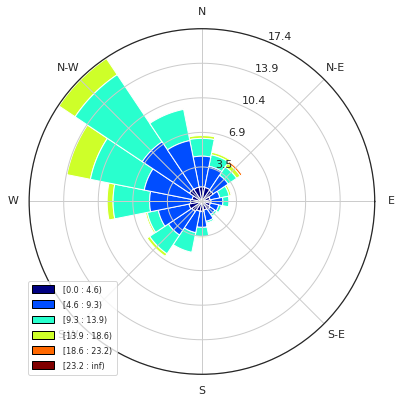## Anomaly¶

Finally, let's show a quick example of an anomaly plot, which depicts the difference in a measured value from a long-term mean. For this example, we'll load approximately 21 years of data and calculate an average temperature for each yearday over the full record. Then we will subtract that average from each day's average to plot the anomaly.

Note, in this example, we also demonstrate how to merge two datasets together, including the archive and recent real-time datasets.

In :
```# Open datasets
ds1 = xr.open_dataset('https://dods.ndbc.noaa.gov/thredds/dodsC/data/stdmet/44025/44025.ncml') #Archive
ds2 = xr.open_dataset('https://dods.ndbc.noaa.gov/thredds/dodsC/data/stdmet/44025/44025h9999.nc') #Realtime

# Subset the dataset
# ds1 = ds1.sel(time=slice('2000-01-01','2020-05-01')) # Set specific date ranges
# ds2 = ds2.sel(time=slice('2020-05-01','2021-01-01'))

ds1 = ds1.where(ds1.time > np.datetime64('2000-01-01'), drop=True) # Grab all data since 1/1/2000
ds2 = ds2.where(ds2.time > ds1.time.max(), drop=True) # Grab all data since the end of the archive

# Convert to Pandas Dataframe
df1 = ds1.to_dataframe().reset_index().set_index('time')
df2 = ds2.to_dataframe().reset_index().set_index('time')

# Merge these datasets
df = df1.combine_first(df2)
```
In :
```# Change plot style again
sns.set_style("darkgrid")

# Plot all data
ds1.sea_surface_temperature.plot()
ds2.sea_surface_temperature.plot();
```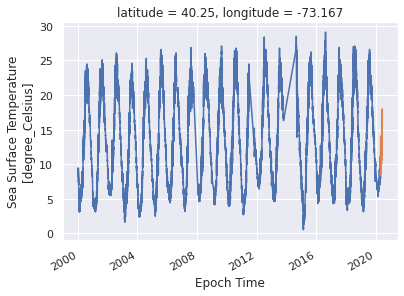In :
```# Anomaly claculation
def standardize(x):
return (x - x.mean())/x.std()

# Calculate a daily average
df_daily = df.resample('1D').mean()

# Calculate the anomaly for each yearday
anomaly = df_daily['sea_surface_temperature'].groupby(df_daily.index.dayofyear).transform(standardize)
```
In :
```# Plot the full record
anomaly.plot();
plt.ylabel('SST Anomaly (C)');
```In :
```# Plot just this year (so far)
anomaly.plot();

import datetime
plt.xlim(datetime.date(2020,1,1),datetime.date(2020,7,1))
plt.ylabel('SST Anomaly (C)');
```Note, the code above was adapted from this example from Ryan Abernathey, which calculates the anomaly for the full record that you input into the function.

If you wanted to calculate a climatic average first (say for 1980-2010), and then use that average to calculate an anomaly from a different dataset (say from 2010-2020), you can try the approach used in this example by Sage.